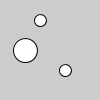Name

### sub()

Examples```v1 = PVector(40, 20, 0)
v2 = PVector(65, 70, 0)

ellipse(v1.x, v1.y, 12, 12)
ellipse(v2.x, v2.y, 12, 12)
v2.sub(v1)
ellipse(v2.x, v2.y, 24, 24)
``````v = PVector(65, 70, 0)

ellipse(v.x, v.y, 12, 12)
ellipse(40, 20, 12, 12)
v.sub(40, 20, 0)
ellipse(v.x, v.y, 24, 24)
``````v1 = PVector(65, 70, 0)
v2 = PVector(40, 20, 0)

ellipse(v1.x, v1.y, 12, 12)
ellipse(v2.x, v2.y, 12, 12)
v3 = PVector.sub(v1, v2)
ellipse(v3.x, v3.y, 24, 24)
```
Description Subtracts x, y, and z components from a vector, subtracts one vector from another, or subtracts two independent vectors. The version of the method that substracts two vectors is a static method and returns a PVector, the others have no return value -- they act directly on the vector. See the examples for more context. In all cases, the second vector (v2) is subtracted from the first (v1), resulting in v1-v2.
Syntax
```.sub(v)
.sub(x, y, z)
.sub(v1, v2)
.sub(v1, v2, target)
```
Parameters
v PVector: any variable of type PVector float: the x component of the vector float: the y component of the vector float: the z component of the vector PVector: the x, y, and z components of a PVector object PVector: the x, y, and z components of a PVector object PVector: the x, y, and z components of a PVector object PVector: the x, y, and z components of a PVector object PVector: PVector in which to store the result

Updated on Mon Sep 21 15:53:25 2020.

If you see any errors or have comments, please let us know.